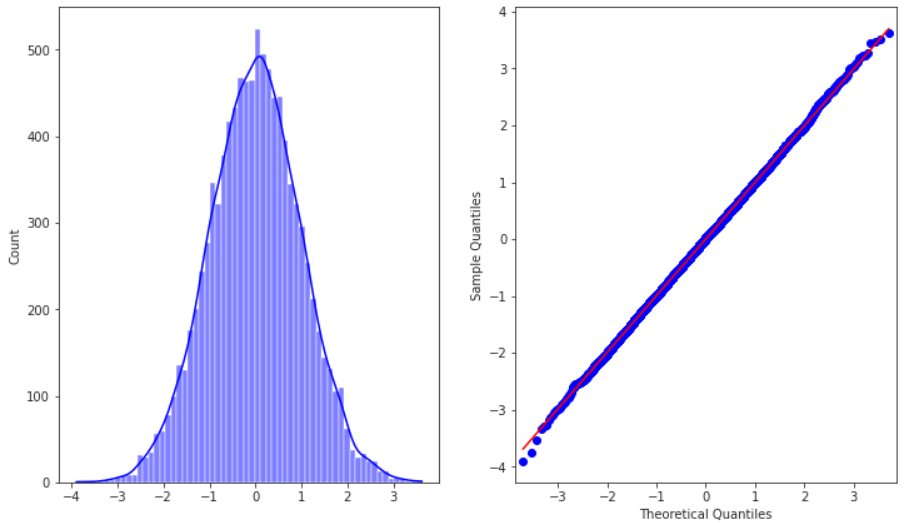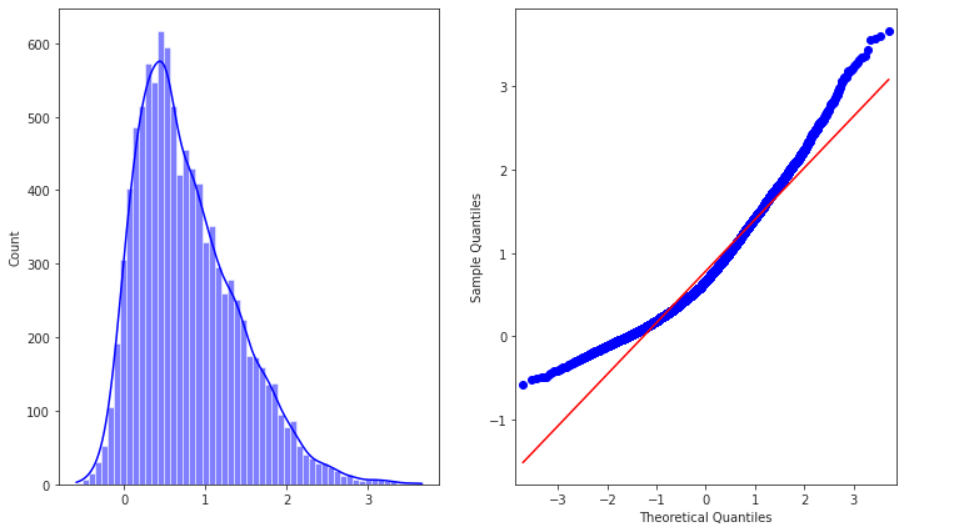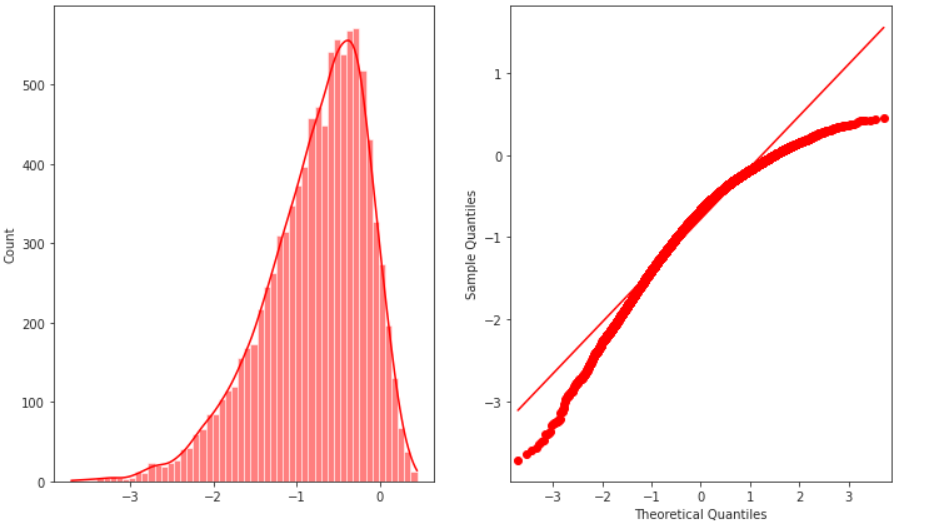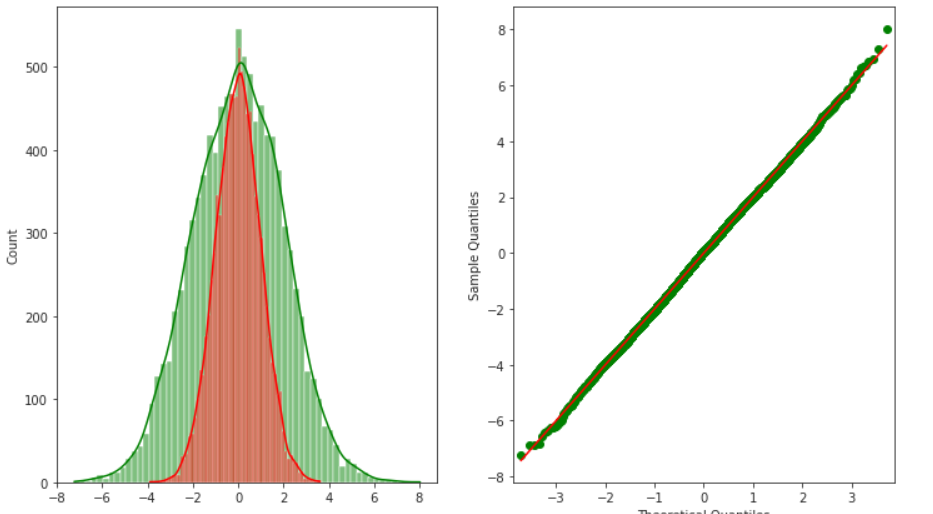# Normal Probability Plot

• Last Updated : 22 Jan, 2021

The probability plot is a way of visually comparing the data coming from different distributions. These data can be of empirical dataset or theoretical dataset. The probability plot can be of two types:

• P-P plot: The (Probability-to-Probability) p-p plot is the way to visualize the comparing of cumulative distribution function (CDFs) of the two distributions (empirical and theoretical) against each other.
• Q-Q plot: The q-q (Quantile-to-Quantile) plot is used to compare the quantiles of two distributions. The quantiles can be defined as continuous intervals with equal probabilities or dividing the samples between a similar way The distributions may be theoretical or sample distributions from a process, etc. The normal probability plot is a case of the q-q plot.

Normal Probability plot: The normal probability plot is a way of knowing whether the dataset is normally distributed or not. In this plot, data is plotted against the theoretical normal distribution plot in a way such that if a given dataset is normally distributed it should form an approximate straight line. The normal probability plot is a case of the probability plot (more specifically Q-Q plot). This plot is commonly used in the industry for finding the deviation from the normal process.

The normal probability plot has the following axis.

• Horizontal Axis: Normal-order statistic medians.
• Vertical Axis: Order response values

The order response variable can be calculated as:Where Ui is the uniform order median statistics and G is the percent point function of normal distribution. It is the inverse of the cumulative distribution function. i.e given probability distribution, we want the cumulative distribution function.

The uniform order statistics medians can be approximated by:The underlying assumptions for a measurement process that the data should have following

• Random numbers.
• From fixed distributions.
• With fixed location
• With a fixed scale.

The normal probability plot is used to answer the following questions:

• Is Data normally distributed?
• If not, what is the nature of distribution

#### Implementation

In this implementation, we will be using statsmodels python library and seaborn library for visualizing different plots. These libraries are pre-installed in colab, but for local environment using pip install.

## Python3

 # imports import numpy as npimport matplotlib.pyplot as pltimport seaborn as snsimport scipy.stats as scimport statsmodels.graphics.gofplots as sm  # define distributionssample_size = 10000 standard_norm = np.random.normal(size=sample_size)heavy_tailed_norm = np.random.normal(loc=0, scale=2, size=sample_size)skewed_norm = sc.skewnorm.rvs(a=5, size=sample_size)skew_left_norm = sc.skewnorm.rvs(a=-5, size=sample_size)    # plots for standard distributionfig, ax = plt.subplots(1, 2, figsize=(12, 7))sns.histplot(standard_norm,kde=True, color ='blue',ax=ax)sm.ProbPlot(standard_norm).qqplot(line='s', ax=ax)  # plot for right-tailed distributionfig, ax = plt.subplots(1, 2, figsize=(12, 7))sm.ProbPlot(skewed_norm).qqplot(line='s', ax=ax);sns.histplot(skewed_norm,kde=True, color ='blue',ax=ax)  # plot for left-tailed distributionfig, ax = plt.subplots(1, 2, figsize=(12, 7))sm.ProbPlot(skew_left_norm).qqplot(line='s',color='red', ax=ax);sns.histplot(skew_left_norm,kde=True, color ='red',ax=ax)  # plot for heavy tailed distributionfig, ax = plt.subplots(1, 2, figsize=(12, 7))sm.ProbPlot(heavy_tailed_norm).qqplot(line='s',color ='green', ax=ax);sns.histplot(heavy_tailed_norm,kde=True, color ='green',ax=ax)sns.histplot(standard_norm,kde=True, color ='red',ax=ax)Standard NormalRight SkewedLeft-SkewedHeavy-tailed (see axis values)

#### References:

My Personal Notes arrow_drop_up